American Journal of Theoretical and Applied Statistics
Volume 5, Issue 2, March 2016, Pages: 58-63

A Comparison of Artificial Neural Network and Time Series Models for Forecasting GDP in Palestine

Samir K. Safi

Dept. of Economics and Statistics, Faculty of Commerce, the Islamic University of Gaza, Gaza, Palestine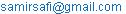Samir K. Safi. A Comparison of Artificial Neural Network and Time Series Models for Forecasting GDP in Palestine. American Journal of Theoretical and Applied Statistics. Vol. 5, No. 2, 2016, pp. 58-63. doi: 10.11648/j.ajtas.20160502.13

Abstract: Time series of quarterly observations on Gross Domestic Product (GDP) is collected and used in this study. Forecasting results of ANNs are compared with those of the Autoregressive Integrated Moving Average (ARIMA) and regression as benchmark methods. Using Root Mean Square Error (RMSE), the empirical results show that ANN performs better than the traditional methods in forecasting GDP.

Keywords: GDP, Artificial Neural Networks, Forecasting, ARIMA, Regression

Contents

1. Introduction

This paper involves an important problem concerning forecasting in time series processes: the construction of time series models to be used for sample forecasting. A good forecasting model is key to proper planning. So most managers take model development seriously, as good planning guide to good and efficient management.

The subject of the present paper is to begin with a given time series that characterizes a natural phenomenon and as usual, is nonstationary. A popular and useful classical procedure to develop forecasting models have been shown to be quite effective . They introduced a procedure for developing a forecasting model that is more effective than the classical approach.

Basic concepts and analytical methods will be reviewed that are essential in structuring the proposed forecasting model. The review is based on the Autoregressive Integrated Moving Average (ARIMA) processes. The classical time series model for the subject information along with the proposed process will be developed.

Economic indicators are the most sensitive sector in any country. Gross Domestic Product (GDP) forecasting is an important issue for governments. Having reliable GDP forecasting information will help the decision makers to predict future values of GDP, then take necessary procedures and yield the required resources to avoid troubles and problems in proposed sector.

In this paper, Artificial Neural Network (ANN), ARIMA and Regression approaches have been conducted for GDP forecasting. The purpose of this paper is to find a simple and reliable forecasting model for the GDP in Palestine. In this paper, the new approach of using ANN will be applied to time series problems and compared to more traditional methods of time series analysis. The expected results are that, comparison of performance between the three models could reveal that ANNs outperform and offer consistent prediction performance compared to ARIMA and regression models, and hence preferable in selecting the most appropriate forecasting model.

The plan for this paper is to build the appropriate regression, ARIMA(p,d,q), and ANN models, The main steps, respectively, are:

Choose the best regression model;

Choose appropriate and estimate ARIMA(p,d,q);

Choose the best ANN model based on several experimental simulations;

Check on the appropriateness of the fitted models and improve it if needed.

For ARIMA model, the overall strategy will first be to decide on reasonable—but tentative—values for p, d, and q. Having done so, we shall estimate the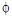’s,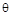’s, and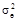for that model in the most efficient way, see for example . Finally, we shall look critically at the fitted model thus obtained to check its adequacy. If the model appears inadequate in some way, we consider the nature of the inadequacy to help us select another model. We proceed to estimate that new model and check it for adequacy.

The time series models such as ARIMA model will be used to find the potential forecasting model. During the calculation process of time series modeling, the Autocorrelation Function (ACF), and the Partial Autocorrelation Function (PACF) criterion will be adopted.

We use a data set of GDP from Palestinian Center of Bureau Statistics (PCBS). The dataset contains the quarterly GDP in Palestine during the period the first quarter of 2000 through the first quarter of 2014. R-statistical software is used for fitting ANN, ARIMA, and regression models for the GDP time series data.

This paper is structured as follows: Section 2 presents the literature review of ANN; the third section demonstrates the comprehensive computer simulation results. This simulation study is designed to compare the forecasting performance of the three dierent models. Section 4 shows the forecasting results using ANN, ARIMA, and Regression models for GDP data; and the last section concludes some important results of this paper and offers future work.

2. Review of ANN Literature

Forecasting models for heat demand a day in advance in a district heating system is presented . The forecasting performance of the models was evaluated based on the generalization performance obtained by cross-validation on a test data set. The main finding is that neural network models with a direct linear link (NNLL) showed the overall best forecasting performance, which suggests that neural network (NN) or the proposed NNLL structures should be considered as forecasting solutions for applied forecasting in district heating markets.

Simulation studies about price modeling via artificial neural networks and proper artificial neural network configurations is constructed . They showed that the neural network model gave better results over a time-series model.

The existence of good model to forecast is very crucial for policy makers. Good policy requires that first identification of relationship for data (linear or non-linear) because it can affect not only housing prices rather all the economy. Research evidence shows that for any system with non-linear instability patterns such as the market for housing, the utilization of the ANN methodology serve properly .

The use of ANNs in economics and finance is a promising field of research especially given the ready availability of large mass of data sets and the ability of ANNs to detect relationships between a large number of variables .

Forecasting the inflow of Dez dam reservoir is introduced . They used Auto Regressive Moving Average (ARMA) and Auto Regressive Integrated Moving Average (ARIMA) models, while increasing the number of parameters in order to increase the forecast accuracy to four parameters and comparing them with the static and dynamic artificial neural networks. By comparing root mean square error (RMSE) and mean bias error (MBE), dynamic artificial neural network model was chosen as the best model for forecasting inflow of the Dez dam reservoir.

The ANN has been used in signal processing due to its nonlinear capacity and robust performance. The structure of the ANN is very important for its performance. For example,  showed that three-layer network is enough to fit any non-stationary signal. In ANN theory, the training data format can affect the performance of network directly. Neural networks are the preferred tool for many predictive data mining applications because of their flexibility, power, accuracy and ease of use.

Additionally,  introduced several non-parametric credit scoring models based on the Multilayer Perceptron approach (MLP) and benchmark their performance against other models which employ the traditional linear discriminant analysis, quadratic discriminant analysis, and logistic regression techniques. Based on a sample of almost 5500 borrowers from a Peruvian microfinance institution, the results reveal that neural network models outperform the other three classic techniques both in terms of area under the receiver-operating characteristic curve and as misclassification costs.

ANN usually uses Back Propagation (BP) as its training algorithm. To improve the performance of the neural network with BP, more training algorithms have been reported in recent years, including Quick Back Propagation (QBP), Resilient Back Propagation (RBP), Broyden – Fletcher – Goldfarb - Shanno Quasi-Newton Back Propagation (BFGS). BGFS algorithm gives the best performance and hence, BGFS algorithm is chosen as the training algorithm of the ANN model .

Furthermore,  introduced two robust forecasting models for efficient prediction of different exchange rates for future months ahead. These models employ Wilcoxon artificial neural network (WANN) and Wilcoxon functional link artificial neural network (WFLANN). Comparison of performance between the two proposed models reveals that both provide almost identical performance but the later involved low computational complexity and hence is preferable over the WANN model.

ANNs constitute one of the most powerful tools for pattern classification due to their nonlinear and non-parametric adaptive-learning properties. Many studies have been conducted that have compared ANNs with other traditional classification techniques, since the default prediction accuracies of ANNs are better than those using classic linear discriminant analysis and logistic regression techniques, see for example  & .

In , Box–Jenkins used statistical models to forecast the financial market. However, the statistical methods assume that data are linearly related and which is typically not true in real life applications. The newly introduced method, ANN, has emerged to be popular as it does not make such assumptions. The ANN, which is inherently a nonlinear network and does not make such assumptions, is well suited for prediction purposes.

3. Simulation Study

3.1. The Simulation Setup

This section presents the comprehensive computer simulation, we consider the substantiality of three forecasting methods: ANNs, ARIMA, and Regression. We compare the ratios of the simulation root mean squared forecast error (RMSFE) of the ANNs model relative to ARIMA and regression as benchmark models.

Three finite sample sizes (30, 50, and 500) and five models of the dependent variable are used; linear, normal, S-curve, logarithmic, and inverse. We also generated a standard normal stochastic design vector of length 10000. We further generated 10000 observations for each of the five dependent variable models. The model coefficients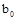, and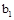were each chosen to be equal one and two, respectively. R functions were used to conduct the simulation.

We introduce deﬁnitions of the simulation RMSFE, the relative efficiency, and the five selected models of dependent variable.

Definition 3.1. The simulation RMSFE,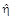, is a measure of the size of the forecast error, that is, the magnitude of a typical mistake made using a forecasting model. The RMSFE is given by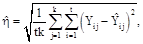where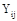denote the i-th actual value of the j-th iteration and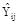denote its forecasted value of the same i and j, t is the sample size (t=50, 100, 500), and k =10000 is the number of simulations. RMSFE is one of the most commonly used measures of forecast accuracy .

Definition 3.2. The efficiency of the ANNs forecasts relative to that of ARIMA in terms of the simulation RMSFE,is given by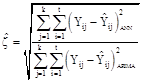A ratio less than one indicates that the ANNs forecast is more efficient than ARIMA, and ifis close to one, then the ANNs forecast is nearly as efficient as ARIMA forecasts. Otherwise, ANNs performs poorly.

Definition 3.3. The models used in the simulation are defined below.

The S-curve model: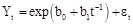The Logarithmic model: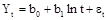The Linear model:The Normal model: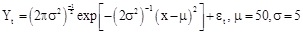The Inverse model: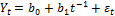The series values are modeled as a function of time t and with independent and identical distributed errors.

3.2. The Simulation Results for RMSFE Estimation

We discuss the simulation results based on the ratio of the estimated RMSFE of ANNs to that of ARIMA and regression. Table (1) shows the complete simulation results for the ratios of RMSFE of ANNs to that of ARIMA and regression for the five different models.

The underlying assumption for the Regression and ARIMA models is that the relationship between the dependent variable and time is linear. So it is not surprising that when we examine the first row of Table (1), we see these methods outperform ANN. Furthermore, the difference in performance is more pronounced when the sample size increases. When the sample size is 500, for example, the Regression is over 40 times more efficient than ANN.

Table 1. Ratios of RMSFE for ANN to ARIMA and Regression.

 Model Sample Sizes 30 100 500 ANN/ARIMA ANN/REG ANN/ARIMA ANN/REG ANN/ARIMA ANN/REG Linear 1.3561 1.5251 5.9849 6.4043 41.2474 41.7027 Normal 0.9670 0.9706 0.9892 0.9864 0.9979 0.9960 S-curve 0.1899 0.1508 0.5643 0.5218 0.7954 0.7831 Logarithmic 0.9132 0.9226 0.9399 0.8200 0.9840 0.7450 Inverse 0.8949 0.9220 0.9530 0.9545 0.9878 0.9858

However, in many real life applications, the underlying model is not linear. So it is important to investigate the robustness of the Regression and ARIMA models to violations of the linearity assumption. Examining the performance of the forecast methods for the other models, we see that the RMSFE ratios are all less than 1, indicating that ANN is the superior method. In most cases, the ratios are relatively close to 1, meaning the gain in efficiency is only moderate in size. The exception is the S-Curve results. For small sample sizes, the ratios are well below 0.200. Another trend, we observe is that in nearly all cases, the ratios increase as the sample size increases. This indicates that the ARIMA and Regression models perform better relative the ANN as the sample size increases, even if the model is not linear. The single exception is the Regression method with the logarithmic model. For this case, the ANN to Regression RMSFE ratio decreases from 0.9226 when t=20 to 0.7450 when t=500, while the ANN to ARIMA RMSFE ratio increases from 0.9132 to 0.9840 for the same sample sizes. So conclude that the Regression method performs poorly when the underlying model is logarithmic.

Overall, for the models selected in this simulation, ANN is the preferable method to use for time series forecasts. ANN outperforms both ARIMA and Regression methods in all models except for the linear model. Furthermore, for the commonly occurring S-Curve model, the difference between ANN and the traditional methods is more pronounced.

4. Fitting Models for GDP Data

This section presents the fitting models for GDP data by using three different approaches, ARIMA(p,d,q), ANN, and Regression models. Consider the quarterly GDP (in billions of USD) in Palestine, from the first quarter in 2000 through the first quarter 2014, the forecasting results are presented in the following sub-sections.

4.1. Fitting ARIMA Model for GDP Data

Figure (1) displays the time series plot. The series displays considerable fluctuations over time, especially in 2002 and 2003, and a stationary model does not seem to be reasonable. The higher values display considerably more variation than the lower values. Note all Figures are shown in the Appendix.

The sample ACF for the data is displayed in Figure (2). All values of the sample ACF for the data are "significantly far from zero," and the only pattern is perhaps a linear decrease with increasing lag. This means that we are dealing with a nonstationary time series. In addition, software implementation of the KPSS test for level stationarity applied to the original GDP leads to a test statistic of 2.6621 and a p-value of 0.01. With stationarity as the null hypothesis, this provides strong evidence supporting the nonstationarity and the appropriateness of taking a difference of the original series.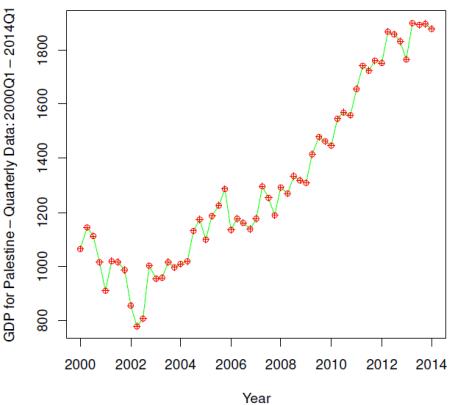Figure 1. Quarterly GDP in Palestine (USD Billion): 2000Q1 – 2014Q1.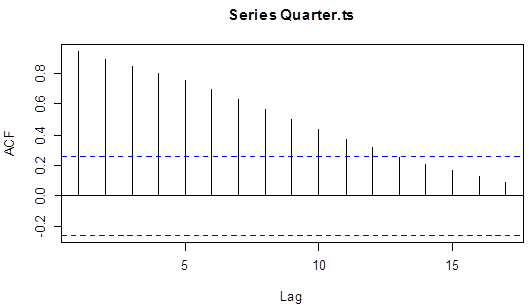Figure 2. Sample ACF for Quarterly GDP.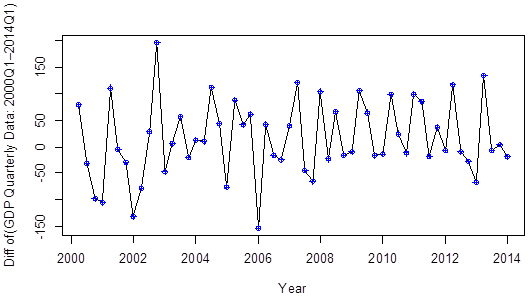Figure 3. The Difference Series of the Quarterly GDP.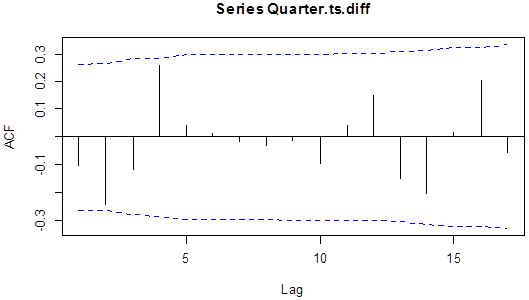Figure 4. Sample ACF for Difference of Quarterly GDP.Figure 5. Sample PACF for Difference of Quarterly GDP.

The differences of the GDP values are displayed in Figure (3). The differenced series of the GDP looks much more stationary when compared with the original time series shown in Figure (1). On the basis of this plot, we might well consider a stationary model as appropriate. KPSS test is applied to the differenced series leads to a test statistic of 0.1766 and a p-value of 0.10. That is, we do not reject the null hypothesis of Stationarity. The sample ACF and PACF for the differences of the GDP values are shown in Figures (4) and (5), respectively. From these figures, it is quite clear the series is white noise, i.e. ARIMA(0,1,0). The estimated model in (1) is fitted by using the maximum likelihood estimation. The estimated noise variance is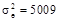.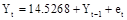(1)

Figure (6) displays the time series plots of the fitted ARIMA (0,1,0) Model from GDP. The plot of the standardized residuals from the ARIMA(0,1,0) model shows only two residuals with absolute magnitude larger than 2. The points of a quantile-quantile plot of the residuals from the ARIMA(0,1,0) seem to follow the straight line fairly closely. This graph would not lead us to reject normality of the error terms in this model. In addition, the Shapiro-Wilk normality test is applied to the residuals produces a test statistic of ks = 0.9821, which corresponds to a p-value of 0.56, and we would not reject normality based on this test. To check on the independence of the error terms in the model, we consider the sample ACF of the residuals. This graph does not show statistically significant evidence of nonzero autocorrelation in the residuals. In other words, there is no evidence of autocorrelation in the residuals of this model. These residual autocorrelations look excellent.

In addition to looking at residual correlations at individual lags, it is useful to have a test that takes into account their magnitudes as a group. The Ljung-Box test statistic with K = 5 is equal to 7.698076. This test is referred to a chi-square distribution with four degrees of freedom. This leads to a p-value of 0.1088858, so we have no evidence to reject the null hypothesis that the error terms are uncorrelated. The suggested model looks to fit the modeling time series very well.Figure 6. Plots of the Fitted ARIMA (0,1,0) Model for Quarterly GDP.

Therefore, the estimated ARIMA(0,1,0) model seems to be capturing the dependence structure of the difference of GDP time series quite well. The ARIMA(0,1,0) result shows that the RMSFE equals 70.99527.

4.2. Fitting ANN Model for GDP Data

Applying ANN with average of 20 networks, each of which is a 1-1-1 network. R-software is used for fitting ANN model for the time series. Some commands and functions with input and output variables have been used. The nnetar function is used to fit neural networks . The estimated noise variance is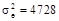. RMSFE is used as stopping criteria in the network. Smaller values of RMSFE indicate higher accuracy in forecasting. The Neural network result shows that the minimum RMSFE equals 68.49194.

4.3. Fitting Regression Model for GDP Data

The estimated linear regression model in (2) is obtained by using the Ordinary Least Square (OLS) estimation method;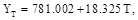(2)

where T is the time. We also see that the estimated noise variance is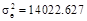. The regression result shows that the RMSFE equals 118.417.

The RMSFE for forecasting using ANN, ARIMA, and Regression equal 68.763, 70.995, and 118.417, respectively. This result shows that RMSFE forecasting of ANN is the smaller than that by using ARIMA and Regression models. This means ANN model for forecasting is more accurate and efficient than the ARIMA and Regression forecasting models.

Table (2) shows the forecasting results for GDP (USD Billion) in 2014Q2-2016Q4 based on ANN, ARIMA (0,1,0), and Regression models.

Table 2. Forecasting results of ANN, ARIMA (0,1,0), and Regression models for GDP (USD Billion) in 2014Q2-2016Q4.

 Year ANN ARIMA Regression 2014-Q2 1885.744 1878.5 1844.14 2014-Q3 1892.076 1878.5 1862.47 2014-Q4 1897.588 1878.5 1880.8 2015-Q1 1902.371 1878.5 1899.13 2015-Q2 1906.507 1878.5 1917.46 2015-Q3 1910.075 1878.5 1935.79 2015-Q4 1913.145 1878.5 1954.12 2016-Q1 1915.783 1878.5 1972.45 2016-Q2 1918.044 1878.5 1990.78 2016-Q3 1919.98 1878.5 2009.11 2016-Q4 1921.635 1878.5 2027.44

5. Conclusions

This paper has proposed three efficient approaches forecasting models. In the first model artificial neural network is used, the second model consists of using ARIMA model, and we consider the time series simple regression model as the third model on real data for GDP in Palestine. Using comprehensive simulations and real data for GDP, the major finding reveals that ANNs outperform the ARIMA and regression models in all models except for the linear model. In addition, ANNs offer consistent prediction performance compared to ARIMA and regression model and hence preferable as a robust prediction model.

Acknowledgment

I would like to express the deepest appreciation to Dr. Alexander White, Department of Mathematics, Texas State University, San Marcos for his valuable comments, suggestions, and reviews. Also, I am grateful for the referees for their valuable comments and on earlier draft of this paper.

References

1. Bahramianfar, P. Forecasting US Home Prices with Neural Network and FuzzyMethods. LAP LAMBERT Academic Publishing, Germany, 2015.
2. Blanco, A., Pino-Mejías, R., Lara, J., Rayo, S. Credit scoring models for the microfinance industry using neural networks: Evidence from Peru. Expert Systems with Applications, 2013, 40(1): 356-364.
3. Box, G. E. P. & Jenkins, G. M. Time series analysis, forecasting and control. San Francisco: Holden-Day, 1976.
4. Box, G. E. P., Jenkins, G. M., & Reinsel, G. C. Time series analysis forecasting and control, Third Edition. New Jersey: Prentice Hall, 1995.
5. Cadenas E. & Rivera W. Short term wind speed forecasting in La Venta, Oaxaca, México, using artificial neural networks. Renew Energy, 2009, 34(1): 274-8.
6. Cryer, J. D. & Chan, K. S. Time series analysis with applications in R, Second Edition. New York: Springer,2008.
7. Hamid, S. A., & Habib, A. Financial Forecasting with Neural Networks. Academy of Accounting and Financial Studies Journal. 2014, 8(4).‏
8. KÖLMEK, M. A., & Navruz, I. Forecasting the day-ahead price in electricity balancing and settlement market of Turkey by using artificial neural networks. Turkish Journal of Electrical Engineering & Computer Sciences, 2015, 23(3).‏
9. Lee, T. S. & Chen, I. F. A two-stage hybrid credit scoring model using artificial neural networks and multivariate adaptive regression splines. Expert Systems with Applications, 2005, 28(4): 743–752.
10. Lee, T. S., Chiu, C. C., Lu, C. J. & Chen, I. F. Credit scoring using the hybrid neural discriminant technique. Expert Systems with Applications, 2002, 23(3): 245–254.
11. Liu, H., Chen, C., Tian, H., & Li, Y. A hybrid model for wind speed prediction using empirical mode decomposition and artificial neural networks. Renewable Energy, 2012, 48: 545-556.
12. Majhi, B., Rout, M., Majhi, R., Panda, G., & Fleming, P. New robust forecasting models for exchange rates prediction. Expert Systems with Applications, 2012, 39: 12658–12670.
13. Potočnik, P., Strmčnik, E., & Govekar, E. Linear and Neural Network-based Models for Short-Term Heat Load Forecasting. Journal of Mechanical Engineering, 2015, 61(9), 543-550.‏
14. Stock, J. and Watson, M. Introduction to Econometrics. Updated third edition. Prentice Hall, 2015.
15. Valipour, M., Banihabib, M. E., & Behbahani, S. M. R. Comparison of the ARMA, ARIMA, and the autoregressive artificial neural network models in forecasting the monthly inflow of Dez dam reservoir. Journal of Hydrology, 2013, 433-441.‏
16. Venables, W. N. and Ripley, B. D. Modern Applied Statistics with S. Fourth edition. Springer, 2002.

 Contents 1. 2. 3. 3.1. 3.2. 4. 4.1. 4.2. 4.3. 5.
Article ToolsAbstractPDF(400K)